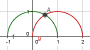# Curve intersection is not defined

Use the command to find the intersection of the curve, the result is not defined

Files: tp.jpg1

Defining the curves in your definition separately as functions, you can see that indeed there's no intersection.

In this case I think working with functions is easier than using curves: f(x)=Function[cos(x), 0, pi]

chris1

hello

there is a bug

saludos

Files: foro.ggb1

1. Intersect[ <Function>, <Function>, <Start x-Value>, <End x-Value> ]

does numeric search so can't always find the intersection

Try

1. f(x) = sqrt(1 - x²)
2. g(x) = sqrt(1 - (x - 1)²)
3. Intersect[f, g, 0, 1]1

yes, it worksbut the help of intersect says

1. Intersect[ <Curve 1>, <Curve 2>, <Parameter 1>, <Parameter 2> ]Finds one intersection point using a numerical, iterative method starting at the given parameters.

Example:

Let a = Curve[cos(t), sin(t), t, 0, π] and b = Curve[cos(t) + 1, sin(t), t, 0, π].

Intersect[a, b, 0, 2] yields the intersection point A = (0.5, 0.87).

saludos1

1. does numeric search so can't always find the intersection

... but in this case it is actually a bug. Fixed for next release (5.0.333.0)